Mathematics

# What are Frequency Tables and Frequency Graphs?

492 views

16 December 2020

## Introduction

What are the different types of graphical representations?

What are frequency distributions and frequency tables?

Graphical representation refers to the use of charts and graphs to visually display, analyze, clarify, and interpret numerical data and other functions.

The principal purpose of a graph is to answer questions about data. Answers to the questions may then provide direction for additional analysis or convey information about the data. It exhibits the relation between data, ideas, information, and concepts in a diagram.

It depends on the type of information in a particular domain. Data on charts is represented by various symbols, such as lines on a line chart, bars on a bar chart, or slices on a pie chart, from which users can gain greater insight than just by numerical analysis.

Representational graphics can illustrate general behavior and patterns, anomalies, and relationships between data points that may otherwise be overlooked, thereby contributing to predictions and better, data-driven decisions. The types of representational graphics used depend on the type of data being explored.

## What are Frequency Tables and Frequency Graphs? - PDF

If you ever want to read it again as many times as you want, here is a downloadable PDF to explore more.

## What are the different types of Graphical Representations?

### Line Graphs

The line graph or the linear graph is used to display continuous data, and it is useful for predicting future events over time. It is used to show information that changes over time. Line graphs are plotted using several points connected by straight lines. It is also called a line chart. The line graph comprises of two axes known as 'x' axis( horizontal) and 'y' axis( vertical)

Example:

The table below shows daily temperatures for New Delhi, recorded for 6 days, in Celsius.

Temperature In New Delhi

Day

Temperature ( in Celsius)

1

38

2

35

3

31

4

29

5

25

6

22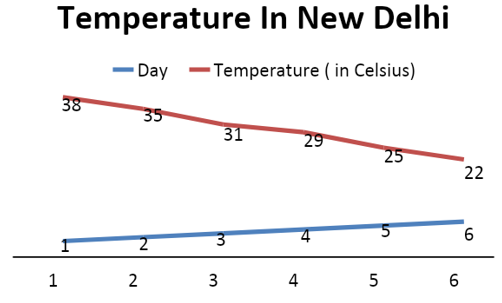In this example, the temperature changed from day to day.

### Bar graph

A Bar Graph or a Bar Chart is a graphical display of data using bars of different heights. It is used to display the category of data, and it compares the data using solid bars to represent the quantities. It is useful for looking at a set of data to make comparisons. A bar graph is the simplest way to represent data.

Example:

The following data gives information of the number of children involved in different activities.

 Activities Dance Music Art Cricket Football No of children 40 55 60 83 90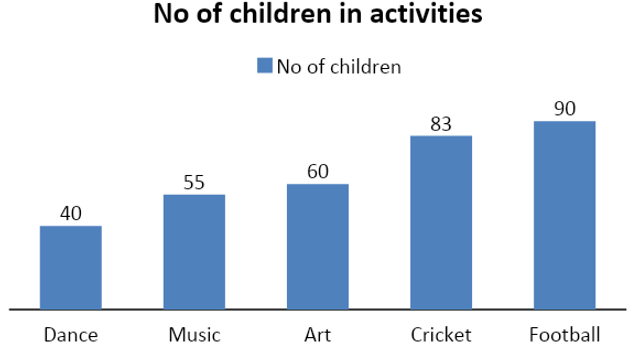### Pictograms

A pictogram is a chart that uses pictures to represent data. Pictograms are set out in the same way as bar charts, but instead of bars, they use columns of pictures to show the numbers involved. They are symbols that convey meaning through its resemblance to a physical object.

Example: The following pictogram shows the number of students using the various modes of transport to go to school.

Each stick figure represents four students.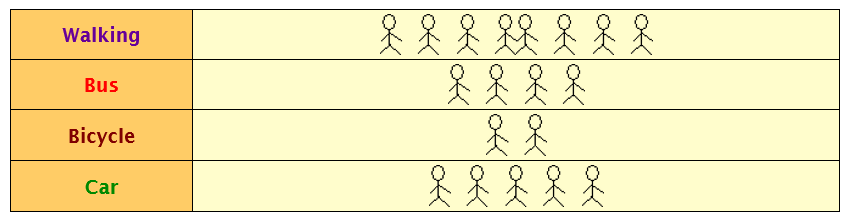Therefore, the numbers of students are as follows:

Walking: 32

Bus: 16

Bicycle: 8

Car: 20

### Pie charts

A Pie Chart displays data in a circular graph. The pieces of the graph are proportional to the fraction of the whole in each category. It is a special chart that uses pie slices to show relative sizes of data. The total value of the pie is always 100%. The values in the table are added to get a total. Each value is then divided by the total and multiplied by 100 to get a percentage.

Example: Survey a few people to find the kind of movie they like best:

 Favorite Type of Movie Mystery Action Thriller Drama War Total 4 5 6 1 4 20 4/20*100 20 5/20*100 25 6/20*100 30 1/20*100 5 4/20*100 20 100%

To represent it on a pie chart, divide each value by the total and multiply by 100 to get a percent.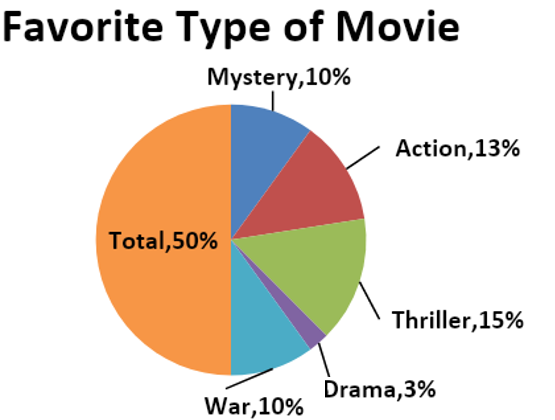### Frequency diagrams and polygons

Data is represented in many different forms. Using frequency diagrams or polygons can make information easier to understand.

For convenience, diagrams produced from frequency tables, whether bar charts or some other kind of chart, are all called frequency diagrams. Bar charts can be drawn with either horizontal or vertical bars, but frequency diagrams often have vertical bars.

A frequency diagram, often called a line chart or a frequency polygon, shows different groups’ frequencies. It is a graph constructed by using lines to join the midpoints of each interval or bin.

The heights of the points represent the frequencies. A frequency polygon can be created from the histogram or by calculating the bins’ midpoints from the frequency distribution table.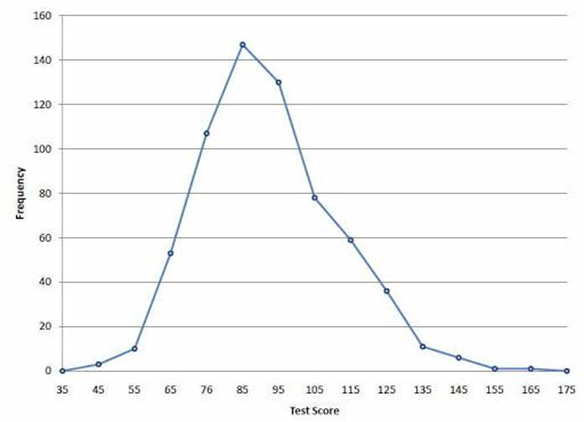### Scatter diagrams

The scatter diagram graphs pairs of numerical data, with one variable on each axis, to look for a relationship between them. If the variables are correlated, the points will fall along a line or curve. The better the correlation, the tighter the points will hug the line.

Scatter plots (also called scatter graphs) are similar to line graphs. A line graph uses a line on an X-Y axis to plot a continuous function, while a scatter plot uses dots to represent individual pieces of data.

Example: The local ice cream shop keeps track of how much ice cream they sell versus the noon temperature on that day. Here are their figures for the last 12 days:

Ice Cream Sales vs. Temperature

Temperature °C

Ice Cream Sales

14.2°

$215 16.4°$325

11.9°

$185 15.2°$332

18.5°

$406 22.1°$522

19.4°

$412 25.1°$614

23.4°

$544 18.1°$421

22.6°

$445 17.2°$408

When plotted as a scatter diagram, it is easy to see that warmer weather leads to more sales.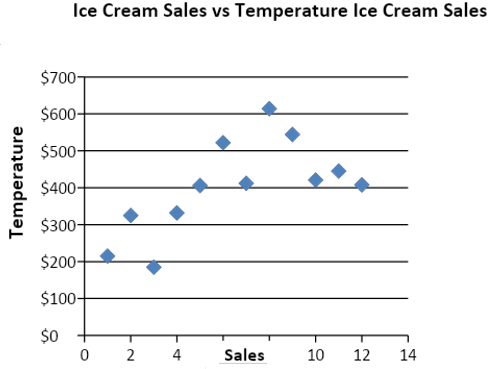## How to track data using Tally Marks?

Tally marks are a quick way of keeping track of numbers in groups of five. One vertical line is made for each of the first four numbers; the fifth number is represented by a diagonal line across the previous four.

When the observations are large, it may not be easy to find the frequencies by simply counting, so we use bass (|, \) called the tally marks. The cluster size 5 has the advantages of easy conversion into decimal for higher arithmetic operations and avoiding error, as humans can far more easily correctly identify a cluster of 5 than one of 10.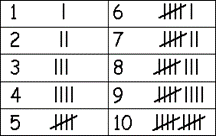## What is Frequency distribution?

Frequency is how often something occurs. By counting frequencies, we can make a Frequency Distribution table.

Example:

Megan owns a bakery. She counts the number of customers she has each day at lunchtime on 30 consecutive days. The results are shown here.

 13 8 16 12 12 16 7 18 11 16 15 7 11 12 13 21 17 19 11 14 10 19 13 12 7 16 6 14 12 18

A grouped frequency table would give an overview of the data. The smallest number is 6, and the biggest number is 21, so groups that have a width of 5 are reasonable. This will give four groups, as shown below. E.g., 5 to 10 customers visit the bakery six times a month. Tally marks are often used to make a frequency distribution table.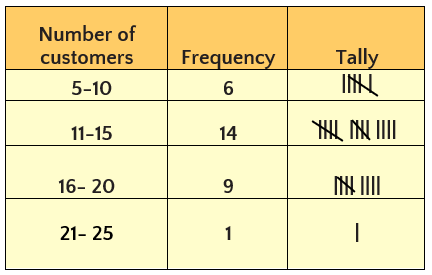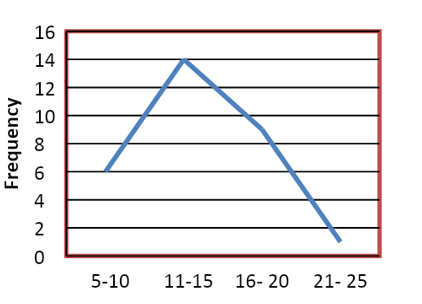## What are Frequency Tables?

A frequency table is a table that lists items and shows the number of times the items occur. We represent the frequency by the alphabet ‘f’.

A frequency table is a method of organizing raw data in a compact form by displaying a series of scores in ascending or descending order. Tally marks help in counting the frequency of occurrence of something. The frequency tables allow us to look at the entire data values in a more ordered and easy manner.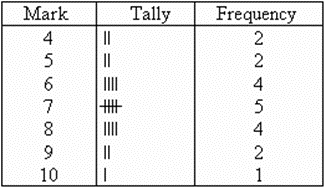## Applications of Frequency Tables in Real Life

Frequency and frequency distribution is the basic building block of statistical analytical methods and the first step in analyzing survey data. It helps researchers to organize and summarize the survey data in a tabular format, (b) interpret the data, and (c) detect outliers (extreme values) in the survey data set.

## Summary

Real-world data can be easily and accurately represented by using various types of graphs, frequency tables, etc. to display the data. As already elaborated upon, frequency tables keep a tally of how often certain events occur. A tally is simply a running total. They are most useful when keeping track of how many times something happens.

Grouping and sorting data can be easily done through frequency distributions and tables. It has great importance in statistics. Also, a well-structured frequency distribution makes it possible to get a detailed analysis of the available data.

Graphs and statistics are essential in any field of work, sports, business, medicine, etc. Master the concepts of statistics and graphs with us, sign up for a free trial.

Cuemath, a student-friendly mathematics and coding platform, conducts regular Online Live Classes for academics and skill-development, and their Mental Math App, on both iOS and Android, is a one-stop solution for kids to develop multiple skills. Understand the Cuemath Fee structure and sign up for a free trial.

## What is data?

Any bit of information that is expressed in a value or numerical number is data. For example, the marks you scored in your Math exam is data, and the number of cars that pass through a bridge in a day is also data. Data is basically a collection of information, measurements, or observations.

## How do you differentiate between data and information?

Data is a raw and unorganized fact that is required to be processed to make it meaningful. Information is processed, organized, and structured data. It provides context for data.

## What are the ways to represent data?

Tables, charts, and graphs are all ways of representing data.

## What are the different types of graphs?

The four most common are line graphs, bar graphs and histograms, pie charts, and Cartesian graphs.

## What does frequency represent?

Frequency is the number of times the observation occurred/recorded in an experiment or study.

## What is the difference between grouped and ungrouped data?

Ungrouped data is raw data. This means that it has just been collected but not sorted into any group or classes. On the other hand, grouped data is data that has been organized into groups from the raw data.

## External References

Frequency Distribution